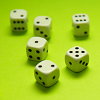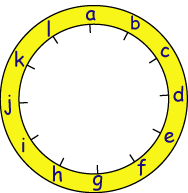#### You may also like### Consecutive Numbers

An investigation involving adding and subtracting sets of consecutive numbers. Lots to find out, lots to explore.### Roll These Dice

Roll two red dice and a green dice. Add the two numbers on the red dice and take away the number on the green. What are all the different possible answers?### Domino Square

Use the 'double-3 down' dominoes to make a square so that each side has eight dots.

# A Mixed-up Clock

## A Mixed-up Clock

There is a clock-face where the numbers have become all mixed up. Can you find out where all the numbers have got to from the ten statements below?

Here is a clock-face with letters to mark the position of the numbers so that the statements are easier to read and to follow.1. No even number is between two odd numbers.
2. No consecutive numbers are next to each other.
3. The numbers on the vertical axis (a) and (g) add to $13$.
4. The numbers on the horizontal axis (d) and (j) also add to $13$.
5. The first set of $6$ numbers [(a) - (f)] add to the same total as the second set of $6$ numbers [(g) - (l)] .
6. The number at position (f) is in the correct position on the clock-face.
7. The number at position (d) is double the number at position (h).
8. There is a difference of $6$ between the number at position (g) and the number preceding it (f).
9. The number at position (l) is twice the top number (a), one third of the number at position (d) and half of the number at position (e).
10. The number at position (d) is $4$ times one of the numbers adjacent (next) to it.

### Why do this problem?

This problem is one that needs logical thinking. At first it might look impossible but if the clues are followed in a suitable order then it only requires simple arithmetic to solve.

### Possible approach

You could begin with a few oral challenges to remind the class of number properties such as odd, even and consecutive.  For example, could they suggest three consecutive odd numbers greater than 50 but less than 70?  How many different sets of consecutive odd numbers are there between 50 and 70?

Share the problem with learners and describe briefly what it entails without reading all the information at first.  Invite pupils to talk in pairs about how they would start the problem.  You could read through the clues in turn, stopping after each to give children chance to discuss what exactly could be learned from it.  Give them time to look through each clue in pairs and decide what can be filled in immediately.  You could draw everyone together to share ideas before giving more time to complete the challenge.

A plenary could give learners the opportunity to explain the steps they took to solve the problem.  Making it clear that the ordering of the information constitutes a systematic approach will help them begin to understand the meaning of being systematic and therefore apply it to future problems.

### Key questions

Can you find a statement that gives you a definite number that you know is right?
Can you now find some more that follow from this one?

### Possible extension

Learners could use the clock-face given to work out some clues for themselves.

### Possible support

Some children may need help reading the clues so this may influence your choice of pairs.